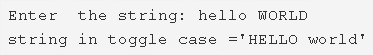# C Program To Toggle Case Of Character Of A String | C Programs

C program to toggle case of each character of a string – In this article, we will describe on the numerous means to toggle case of each character of a string in C programming.

The means explained in this piece are as follows:

• Using Standard Method
• Using Function
• Using Recursion
• Using Pointers Concept

A string is nothing but an array of characters. The value of a string is determined by the terminating character. Its value is considered to be 0.As you can see in the image uploaded above, you need to enter the string that you want to.

The string entered here is “hello WORLD”.

As per the title, the case of the string needs to be altered or toggled.

So, the string after toggling the case is as follows:

“HELLO world”

Thus, the various means to do so in C programming are as follows:

## Using Standard Method

1. Read the entered string and save in the character array s[] using gets(s) function.

2) Now convert the small letter character into a capital letter and vice-versa.

For loop iterates through the string with the structure for(i=0;s[i];i++)

a) If the ASCII value of the character s[i] is in between 65 to 90 then convert the character into the small letter by adding 32 to its ASCII value.

b) If the ASCII value of the character s[i] is in between 97 to 122 then convert the character into the capital letter by subtracting 32 from its ASCII value.

Repeat the loop until the last character of the string becomes to null.

3) Print the string.

Output:

### Using Function

1. The main() function calls the stringtogglecase(char *s) to convert capital letter characters into a small and small case to capital.

2) The function converts the cases of all characters of the string.

The for loop iterates through the string until the last character of the string becomes to null.

a) Check the ASCII value of each character of the string.

b) If the value is in between 65 to 90 then convert the character into the small case by adding 32 to its ASCII.

c) If the value is in between 97 to122 then convert the character into the capital letter by subtracting 32 from its ASCII.

Output:

#### Using Recursion

1. The recursive function calls itself by increasing i value until the character of the string becomes to null.

2) The function checks the ASCII value of the first character of the string.

If it is a small letter then convert into the capital letter by subtracting 32 from its ASCII value.

If it is in a capital letter then convert into the small letter by adding 32 to its ASCII value.

Increase the i value for next character of the string.

The function calls itself(go to the step 2).

Output:

##### Using Pointers Concept
1. p is the pointer variable, it points the character array s[].

2) i=0, the while loop checks the condition if the character at the address of the p[i] is not null then

a) If the ASCII value of the character at the pointer variable p[i] is in between 65 to 90 then convert the Character into the small letter by adding 32 to the ASCII value of the character at the pointer variable p[i].

If the ASCII value is in between 97 to 122 then subtract 32 from the ASCII value of the character at the pointer variable p[i].

b) Increase the i value for next pointed character(go to step 2).

Output:
x

## C Program Inverted Pyramid Star Pattern | 4 Ways – C Programs

C Program to print an inverted pyramid star pattern – In this article, we will ...